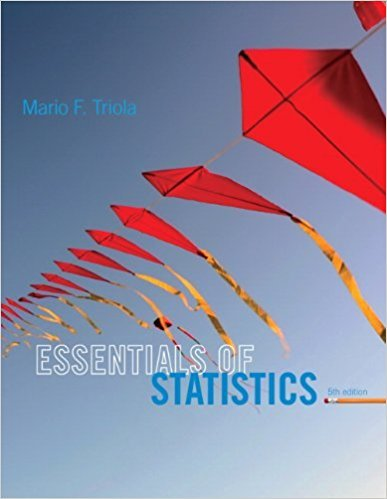×
×

# Solutions for Chapter 10: Correlation and Regression## Full solutions for Essentials of Statistics | 5th Edition

ISBN: 9780321924599Solutions for Chapter 10: Correlation and Regression

Solutions for Chapter 10
4 5 0 337 Reviews
18
2
##### ISBN: 9780321924599

Chapter 10: Correlation and Regression includes 10 full step-by-step solutions. Since 10 problems in chapter 10: Correlation and Regression have been answered, more than 15008 students have viewed full step-by-step solutions from this chapter. This expansive textbook survival guide covers the following chapters and their solutions. Essentials of Statistics was written by and is associated to the ISBN: 9780321924599. This textbook survival guide was created for the textbook: Essentials of Statistics, edition: 5.

Key Statistics Terms and definitions covered in this textbook

A variation of the R 2 statistic that compensates for the number of parameters in a regression model. Essentially, the adjustment is a penalty for increasing the number of parameters in the model. Alias. In a fractional factorial experiment when certain factor effects cannot be estimated uniquely, they are said to be aliased.

• Attribute control chart

Any control chart for a discrete random variable. See Variables control chart.

• Axioms of probability

A set of rules that probabilities deined on a sample space must follow. See Probability

• Bivariate normal distribution

The joint distribution of two normal random variables

• Causal variable

When y fx = ( ) and y is considered to be caused by x, x is sometimes called a causal variable

• Center line

A horizontal line on a control chart at the value that estimates the mean of the statistic plotted on the chart. See Control chart.

• Central limit theorem

The simplest form of the central limit theorem states that the sum of n independently distributed random variables will tend to be normally distributed as n becomes large. It is a necessary and suficient condition that none of the variances of the individual random variables are large in comparison to their sum. There are more general forms of the central theorem that allow ininite variances and correlated random variables, and there is a multivariate version of the theorem.

• Completely randomized design (or experiment)

A type of experimental design in which the treatments or design factors are assigned to the experimental units in a random manner. In designed experiments, a completely randomized design results from running all of the treatment combinations in random order.

• Confounding

When a factorial experiment is run in blocks and the blocks are too small to contain a complete replicate of the experiment, one can run a fraction of the replicate in each block, but this results in losing information on some effects. These effects are linked with or confounded with the blocks. In general, when two factors are varied such that their individual effects cannot be determined separately, their effects are said to be confounded.

• Contingency table.

A tabular arrangement expressing the assignment of members of a data set according to two or more categories or classiication criteria

• Correlation

In the most general usage, a measure of the interdependence among data. The concept may include more than two variables. The term is most commonly used in a narrow sense to express the relationship between quantitative variables or ranks.

• Curvilinear regression

An expression sometimes used for nonlinear regression models or polynomial regression models.

• Designed experiment

An experiment in which the tests are planned in advance and the plans usually incorporate statistical models. See Experiment

• Discrete random variable

A random variable with a inite (or countably ininite) range.

• Distribution function

Another name for a cumulative distribution function.

• Enumerative study

A study in which a sample from a population is used to make inference to the population. See Analytic study

• Error mean square

The error sum of squares divided by its number of degrees of freedom.

• Error of estimation

The difference between an estimated value and the true value.

• Fraction defective control chart

See P chart

• Frequency distribution

An arrangement of the frequencies of observations in a sample or population according to the values that the observations take on

×